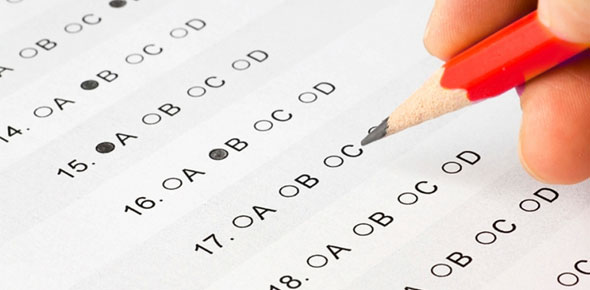# Standardized Assessments

12 Questions | Total Attempts: 80SettingsDirections: Select the best answer for each question. For the extended response question you may type your answer directly in the space provided. After you click "next" for each question you cannot go back and change your response. Make sure you type your name on this page so you get credit for taking it! Scoring: Selected Response: 1 point each question, 10 points total Extended Response: 5 points

Related Topics
• 1.
These assessments compare and individual student's performane against other students who took the test.
• A.

Criterion referenced

• B.

Informal

• C.

Norm referenced

• D.

Performance

• E.

Diagnostic

• 2.
All of these are characteristics of standardized assessments EXCEPT...
• A.

• B.

Often multiple choice

• C.

Usually teacher-created

• D.

Objective scoring

• E.

Allow accommodations for students with IEPs

• 3.
Formal assessments can be used to...
• A.

Evaluate a school district's performance

• B.

Compare students across schools

• C.

Determine eligibility for special education

• D.

Predict a student's performance in the future

• E.

All of the above

• 4.
This type of validity would relate to how well a 10th grade science test actually measures what was covered in class.
• A.

Predictive

• B.

Construct

• C.

Basal

• D.

Content

• E.

Criterion

• 5.
If a student misses 5 math problems in a row on a standardized assessment, the examiner needs to stop the test. This is an example of...
• A.

The student's ceiling level

• B.

Content validity

• C.

Test-retest reliability

• D.

The student's basal level

• E.

The student's cut score

• 6.
For the group of scores: 8, 9, 3, 5, 6, 8, 2, 12, 8 the number 8 would be the
• A.

Mean

• B.

Median

• C.

Mode

• D.

All of the above

• E.

Both b and c

• 7.
In the normal distribution ____% of student would fall between -1 and +1 standard deviation away from the mean.
• A.

34%

• B.

2.0%

• C.

0.5%

• D.

13.5%

• E.

68%

• 8.
Which of these is NOT a type of accommodation?
• A.

Presentation

• B.

Content

• C.

Response

• D.

Timing

• E.

Scheduling

• 9.
If a 2nd grade student receives a GE of 5.5 on a math test, it means
• A.

The student should be in 5th grade

• B.

The student performed as well as a student in the 5th grade, 5th month would do on that test

• C.

The student is as smart as a 5th grade, 5th month student

• D.

The student is 5.5 times better than the average 2nd grader

• E.

The student is in the 55th percentile for 2nd grade

• 10.
A student can only receive an accommodation on a standardized test if it is...
• A.

In the student's IEP

• B.

Approved by the test creators

• C.

Something the student has used on classroom assessments

• D.

All of the above

• E.

All except B

• 11.
Tyesha, a 7th grade student in your class, just took a standardized assessment and received the score report below.  Her mother emails you asking you to explain what the different numbers mean.  Write a short email response to her explaining the different terms and what they say about Tyesha’s ability.  (Scoring:  one point for explanation of each score, one point for comment on what the test says about Tyesha’s academic ability)   Tyesha’s Standardized Test Scores for Math   Standard score                                             182 Grade equivalent                                           5.5 Percentile Rank (district normed)            43 Stanine                                                             5
• 12.
EXTRA CREDIT: List up to three ways that this test does not follow best practices for selected and/or extended response items.  Explain what is wrong with each item you select.  If you would like to look over the test questions again in order to answer this question you can just skip this question for now.  The program will then show you your results on the quiz and will display all of the questions.  You can write up your response for the extra credit on a piece of paper and give it to me in class.  (1 point each, up to 3 extra points)